Next: locally Up: Definitions Previous: light

# like

If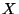is a metric space, a mapping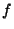fromto a space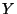is an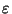-map if, for each point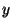of,. If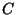is a collection of continua, a continuum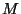is-like if, for every positive number, there exists an-map ofonto an element of. In particular, a continuum is tree-like if, for some collectionof trees,is-like. A concept of a tree-like continuum can be defined in several (equivalent) ways. One of them is the following. A continuumis said to be tree-like provided that for each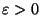there is a tree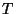and a surjective mapping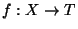such thatis an-mapping (i.e.,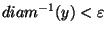for each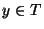). Let us mention that a continuumis tree-like if and only if it is the inverse limit of an inverse sequence of trees with surjective bonding mappings. Compare e.g. [Nadler 1992, p. 24]. Using a concept of a nerve of a covering, one can reformulate the above definition saying that a continuumis be tree-like provided that for eachthere is an-covering ofwhose nerve is a tree.

Finally, the original definition using tree-chains can be found e.g. in Bing's paper [Bing 1951, p. 653].Next: locally Up: Definitions Previous: light
Janusz J. Charatonik, Pawel Krupski and Pavel Pyrih
2001-11-30